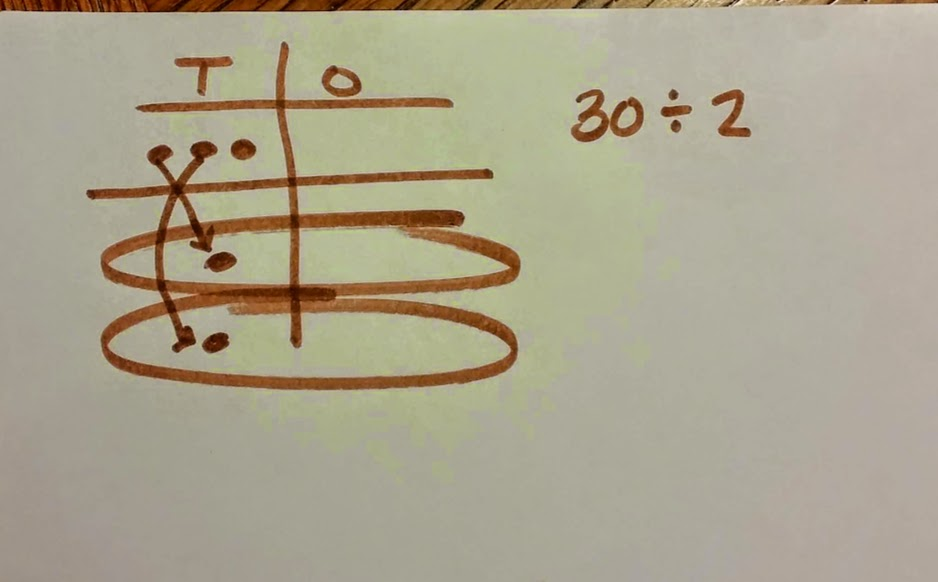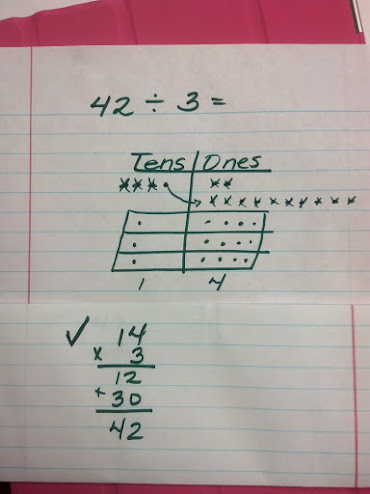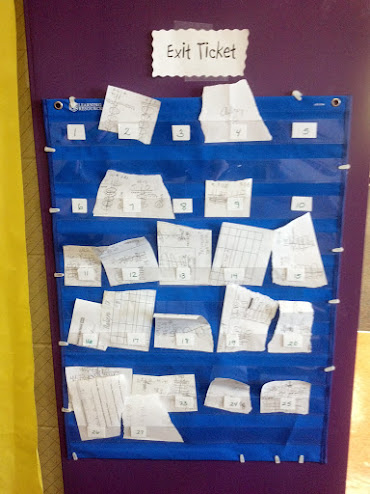# Dividing with Place Value Chart - Decomposing Tens

6 teachers like this lesson
Print Lesson

## Objective

SWBAT represent and solve division problems using a place value chart.

#### Big Idea

In this lesson, students deepen their understanding of division by using an equal distribution method of dividing with a place value chart.

## Warm Up

7 minutes

Students will start today's lesson with a fluency assessment.  This assessment is from Monitoring Basic Skills Progress Second Edition: Basic Math Computation by Lynn S. Fuchs, Carol L. Hamlett, and Douglas Fuchs.

This is an assessment I have my students do each week and then graph their results.  It allows them to reflect on their learning of basic math facts, as well as using all four operations with whole numbers, and adding and subtracting unit fractions.  (It also happens to be the quietest time in my math classroom all week!!)

This is what my classroom looks like as students work on this assessment.

This is a sample of a student's graph.  Please check out the resources to hear my thoughts about students fluency progress.   Since students began the fourth grade set today, I anticipate students graphs to decrease in the number corrected and attempted.

Click hereto see an example of a typical fourth grade fluency decrease now that students are completing the fourth grade fluency set that incorporates division, multiplication, addition, subtraction, and adding and subtracting fractions with like denominators.

Students began the fourth grade set last week.  I do not start my students with the fourth grade skills. I chose to start them with the end of the third grade skills which covers addition, subtraction and multiplication and division of basic facts. I strongly believe in a balanced math approach, which is one reason why I also believe in common core standards.  By having a balance of building conceptual understanding, application of problems, and computational fluency, students can experience rigorous mathematics.  I want to make clear that this assessment ONLY measures basic math computation.  It is only one piece of students' knowledge. The assessments in this book, for each grade level, do not change in difficulty over the course of the year.  Therefore, a student's increase in score over the school year truly reflects improvement in the student's ability to work the math problems at that grade level.

## Concept development

40 minutes

I begin this lesson by displaying 3 ÷ 2 on the board. Students draw a place value chart in their math notebooks, and model this problem with dots or tallies in their chart.  Then I record 3 ÷ 2 as long division as well so students continue to gain familiarity with how long division looks and and can begin to make connections between the model and the algorithm. I encourage students to share relationships between their model and the algorithm they observe.  I ask students what 3 tens divided by 2 is? Distribute your dots/tallies and cross off what what’s been distributed. Many students will respond with 1 ten with a remainder of 1 ten. Next, I model how to decompose 1 ten for 10 ones and then distribute the ten ones to the two groups.

Next, I ask students to write 30 ÷ 2 in their notebooks and model 30 in a place value chart in their notebooks. Many students will respond that they don't need to model this because they already know 30 ÷ 2 = 15.  I remind students that when we are learning a new skill, it is often easier to learn the skill with numbers that are familiar to us first, before we try the skill with unfamiliar numbers and division problems.

I ask students to think about and discuss with their learning partner why we didn’t stop when we had a remainder of 1 ten?  I explicitly state to students since the ones are the smallest unit on our place value chart, we stop there and make a remainder.

Click here to hear me explain what I mean by a remainder of "ten" to help better clarify this lesson and strategy.Below is another example of how I draw the model to show decomposing a ten.For the rest of the class period, students work on problems like these in their math notebooks. 4 ÷ 3, 42 ÷3, 84 ÷ 3, 73 ÷ 2, 56 ÷ 4, 92 ÷ 4  The problems in this practice only have remainders in the tens place that students decompose into tens. I chose to break my lessons using division and a place value chart into several lessons in which students spend significant amounts of time practicing one skill before I add in more complex division problem with remainders in both the tens place and ones place.  This allows students time to build conceptual understanding about division. The CCSS stress not only procedural skill but also conceptual understanding, to make sure students are learning and absorbing the critical information they need to succeed at higher levels - rather than the current practices by which many students learn enough to get by on the next test, but forget it shortly thereafter, only to review again the following year.  Students use Math Practice Standard 7 when they make connections between division and multiplication, but the Math Practice Standard that was this lesson's focus was Practice Standard 4, modeling with mathematics.  Students use the place value model to divide and make connections between concepts through out this lesson.

This student explains the place value chart to divide at the smart board for the class.

Note: For my students working above grade level (only a few) I encourage them to also solve without place value charts to become more efficient at solving long division problems. I also allow them to share and explain their method with others as time permits.

## Student debrief - Wrap up

5 minutes

For this student debrief, I chose to do an exit ticket to make sure I have a pulse of the class' learning of using a place value chart to divide.  The next lesson will entail using a place value chart to divide numbers that require decomposing tens and remainders in the ones. It will be critical for me to know which students are struggling with decomposing tens before they can be successful in the next lesson.

Students use a post-it note and solve 45 ÷ 4 and 48 ÷ 3 using a place value chart. They place in the exit slip holder on the door as the leave the classroom.Click here to see and hear my thoughts about this student's learning.

Click here to see and hear about another student's response.